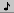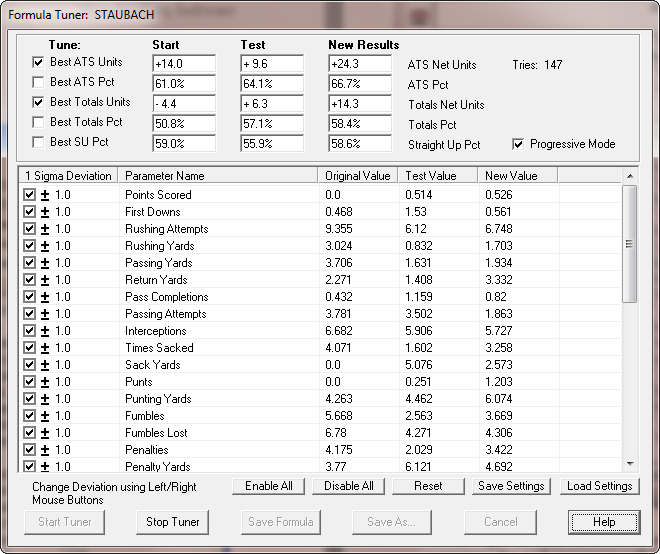## Section 8.5. Tuning a Prediction Formula for Best Results

The Formula Tuner can tune any prediction formula and improve the results. You can start the Formula Tuner from the MY FORMULAS page, using the link called "Tune" to the right of each formula name. You can also select FORMULA TUNER from the FORMULAS menu to tune the active formula, or click thebutton on the toolbar.

Most of the formula equations in WinPicks are non-linear. Finding a mathematical solution for optimizing a formula is difficult (if not impossible). WinPicks Pro Football Analyst has a mathematical tool for optimizing formulas called Surelock which can work with up to 10 parameters. However, in many cases the Formula Tuner will produce better formulas than Surelock does, since it is adjusts all parameters. Plus, it works for all sports, including college football, and pro and college basketball.

Instead of using mathematical equations to solve for the best combination of formula values, the Tuner uses a Monte Carlo technique that randomly varies the numbers, testing the results along the way. When a finds a better result, it remembers the new formula values, and continues to run with new random variations. The "Formula Tuner" runs continuously under your control, letting you tune a formula for a few minutes, a few hours, or even overnight. The "Formula Tuner" never gets tired, and continually tries to make a formula better. It can start with any formula, and in nearly all cases produce a better one.

### The Formula Tuner Window

The Formula Tuner window is shown in Figure 8.50 below:Figure 8.50 - The Formula Tuner Window

At the top of the "Formula Tuner" window, you can select the type of results you wish to tune. You can select one or more of five different options:

• Best ATS Units - Tunes a formula to produce the highest number of against the spread net units

• Best ATS Pct - Tunes a formula to produce the highest winning percentage against the spread. If the tuner reaches 100%, it will continue to try to increase the number of net units.

• Best Totals Units - Tunes a formula to produce the highest number of net units against the totals line (over/unders)

• Best Totals Pct - Tunes a formula to produce the highest winning percentage for over/unders

• Best SU Pct - Tunes a formula to produce the highest percentage of straight-up winners

Listed to the right, there are three columns that show the results of testing the formula. The original results of the formula (before tuning) are displayed in the first column. The second column displays the results of the last test that the Formula Tuner attempted. The numbers in the second column change as the Tuner runs, on many computers a test lasts less than 1 second. The third column shows the results of the best formula the the Tuner has found since it started running.

The "Progressive Mode" checkbox on the window tells the Tuner to add random variations to the best formula the tuner has found so far. If the "Progressive Mode" checkbox is not checked, the Tuner will always add random variation to the original formula every time. The progressive mode is turned on by default, and allows the "Formula Tuner" to "walk" away from the original formula values as it finds better combinations.

In the middle of the "Formula Tuner" window, every parameter in the formula is shown along with its starting value, the last value the tuner checked, and the new value from the best formula found so far.

The first column of the parameter window, labeled "1 sigma deviation", contains a checkbox which lets you to enable or disable each parameter from being tuned. By default all parameters are enabled, but you can disable a parameter if you do no want the value to change. You can enable or disable parameters before starting the "Formula Tuner" or while it is running.

To the right of each checkbox is a number indicating how many standard deviations the random variations for each parameter will be allowed to wander. The "Formula Tuner" generates random variations using a normal distribution within the one sigma (one standard deviation) range. However, while the tuner is running, you can use the left and right mouse buttons to increase and decrease the size of the range where the random variations will be allowed to occur.

There are a row of five buttons directly underneath the formula parameters window:

• Enable All - This button enables all formula parameters to be tuned, including those that were previously disabled.

• Disable All - This disables all parameters from being tuned, allowing you to turn all parameters off, and then turn on the ones you want, one by one using the checkboxes.

• Reset - This button changes all of the sigma values back to their standard value of one (one standard deviation).

• Save Settings - Clicking this button allows you to save the current formula tuner settings in a file. You are allowed to name the file, and store as many tuner parameter files as you wish. The formula settings that you save can also be used later with the NightShift Formula Tuner.

• Load Settings - Click this button to load a previously saved parameter file into the Formula Tuner.

To start tuning, click the "Start" button at the bottom of the window. Once running, you will see the results in the "Test Value" column update as the tuner searches for a better formula, and the number of tries is also updated in the window.

You can click the "Stop" button at any time to stop the tuner from running, and save the formula with the new values. The "Save" button will save the formula with the same name as before, or you can click the "Save As" button to save the formula with a new name.

After saving a formula, you can click the "Cancel" button to leave the "Formula Tuner" and to begin using your new formula.

Note that WinPicks will allow you to run multiple copies of the "Formula Tuner" by launching multiple copies of WinPicks. This allows you to get the most out of your available processing power. For example, you can launch four copies of College Basketball Analyst (CBA) with each one tuning a different formula. Or, you can tune formulas with the college and pro football versions of WinPicks simultaneously, with both tuners running at high speed.